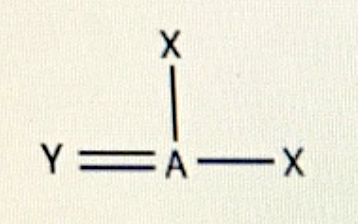# Problem: What would you expect to be the electron-group arrangement around atom A in the following case? Give the ideal bond angle. Electron-group arrangement:a. trigonal bipyramidalb. square planarc. trigonal planarIdeal bond angle:  ____°

###### FREE Expert Solution
90% (172 ratings)
###### FREE Expert Solution
90% (172 ratings)###### Problem Details

What would you expect to be the electron-group arrangement around atom A in the following case? Give the ideal bond angle.Electron-group arrangement:

a. trigonal bipyramidal

b. square planar

c. trigonal planar

Ideal bond angle:  ____°

What scientific concept do you need to know in order to solve this problem?

Our tutors have indicated that to solve this problem you will need to apply the Bond Angles concept. You can view video lessons to learn Bond Angles. Or if you need more Bond Angles practice, you can also practice Bond Angles practice problems.

What is the difficulty of this problem?

Our tutors rated the difficulty ofWhat would you expect to be the electron-group arrangement a...as medium difficulty.

How long does this problem take to solve?

Our expert Chemistry tutor, Dasha took 1 minute and 23 seconds to solve this problem. You can follow their steps in the video explanation above.

What professor is this problem relevant for?

Based on our data, we think this problem is relevant for Professor Stankus' class at UIW.